# Solved Problems on Transistor

### Solution :

Fig.1 shows the conditions of the problem. Here the output resistance is very high as compared to input resistance, since the input junction (base to emitter) of the transistor is forward biased while the output junction (base to collector) is reverse biased.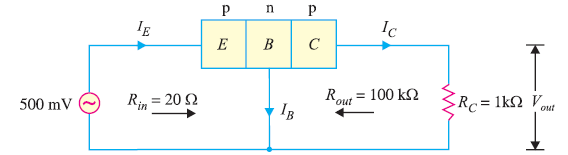Fig. 1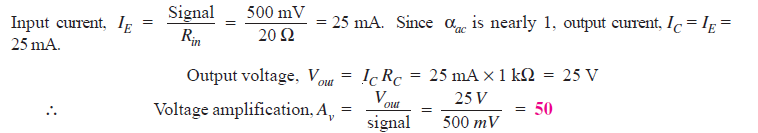### Solution :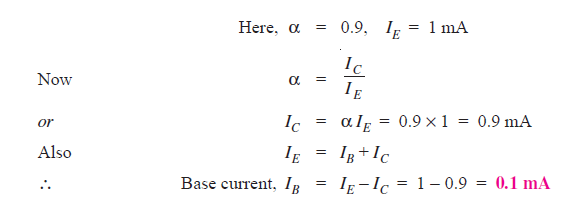### Solution :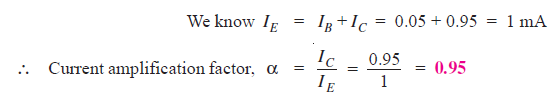### Solution :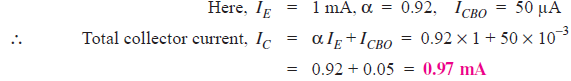### Solution :

Fig. 2 shows the required common base connection.

The voltage drop across RC (= 2 kΩ) is 2V.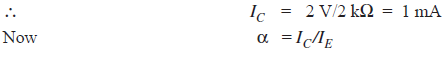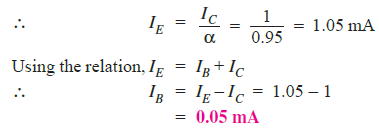### Solution :

Since the transistor is of silicon, VBE = 0.7V.

Applying Kirchhoff’s voltage law to the emitter-side loop,we get,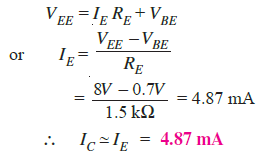Applying Kirchhoff’s voltage law to the collector-side loop, we have,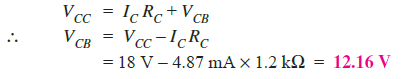### Solution :

(i) α = 0.9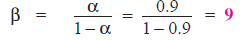(ii) α = 0.98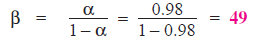(iii) α = 0.99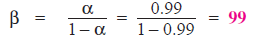### Q10. Find the α rating of the transistor shown in Fig. 4. Hence determine the value of IC using both α and β rating of the transistor.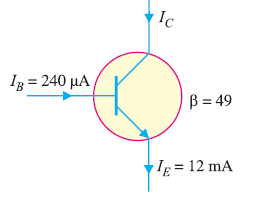Fig. 4

### Solution :

Fig. 8.20 shows the conditions of the problem.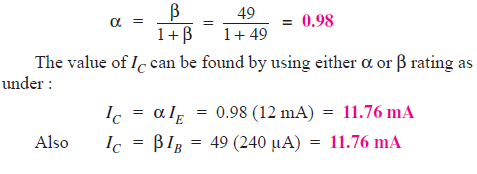### Solution :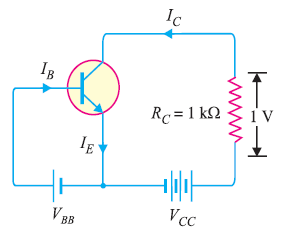Fig. 5

Fig. 5 shows the required common emitter connection. The voltage drop across RC (= 1 kΩ) is 1 volt.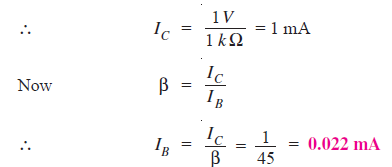### Solution :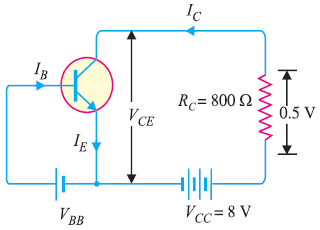Fig.6

Fig. 6 shows the required common emitter connection with various values.

(ii)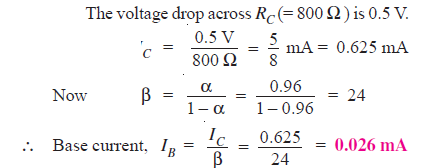### Solution :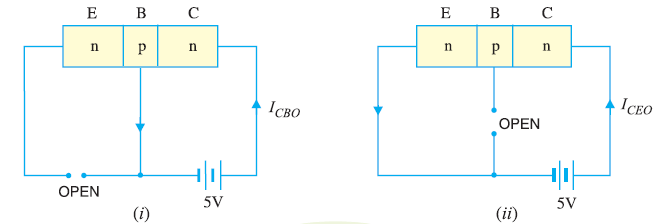Fig. 7

When the emitter circuit is open as shown in Fig.7 (i) , the collector-base junction is reverse biased. A small leakage current ICBO flows due to minority carriers.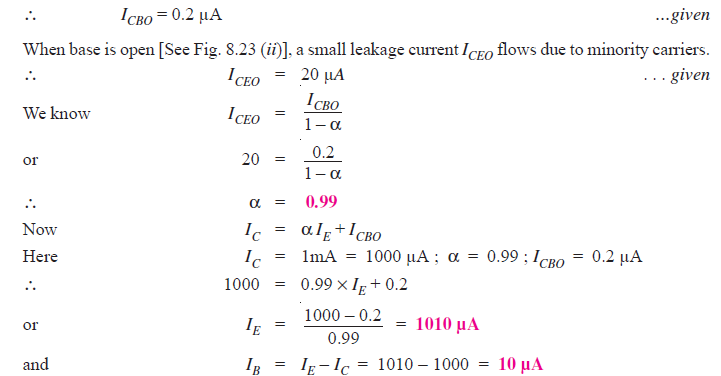### Solution :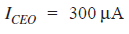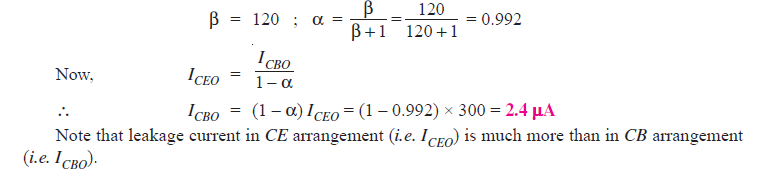### Solution :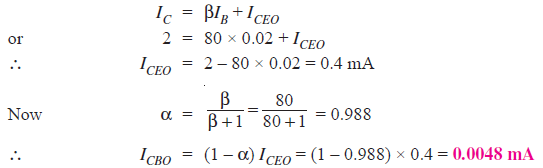### Solution :

The leakage current ICBO is the current that flows through the base-collector junction when emitter is open as shown is Fig. 8.Fig. 8

When the transistor is in CE arrangement, the base current (i.e. ICBO) is multiplied by β in the collector as shown in Fig. 9.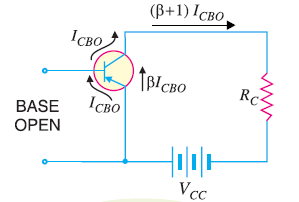Fig.9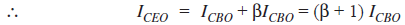### Solution :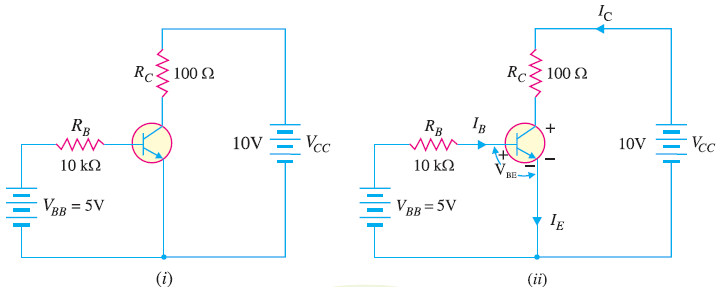Fig.10

Fig. 10 (i) shows the transistor circuit while Fig. 10 (ii) shows the various currents and voltages along with polarities.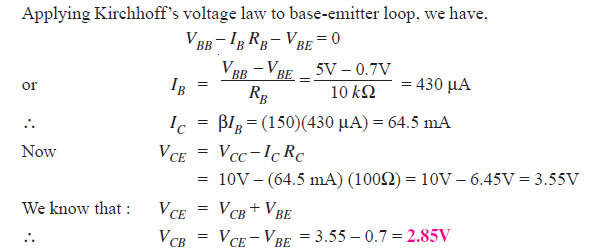### Solution :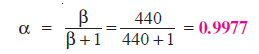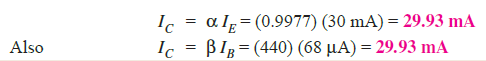### Solution :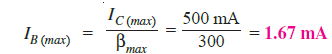For this transistor, if the base current is allowed to exceed 1.67 mA, the collector current will exceed its maximum rating of 500 mA and the transistor will probably be destroyed.

### Solution :Fig. 11

Fig 11 shows the open circuit failures in a transistor. We shall discuss the circuit behaviour in each case.

(i) Open emitter :

Fig. 11 (i) shows an open emitter failure in a transistor. Since the collector diode is not forward biased, it is OFF and there can be neither collector current nor base current.
Therefore, there will be no voltage drops across either resistor and the voltage at the base and at the
collector leads of the transistor will be 12V.

(ii) Open-base :

Fig. 11 (ii) shows an open base failure in a transistor. Since the base is open, there can be no base current so that the transistor is in cut-off. Therefore, all the transistor currents are 0A. In this case, the base and collector voltages will both be at 12V.

(iii) Open collector :

Fig. 11 (iii) shows an open collector failure in a transistor. In this case, the emitter diode is still ON, so we expect to see 0.7V at the base. However, we will see 12V at the collector because there is no collector current.

### Q21. For the circuit shown in Fig. 12 , draw the d.c. load line.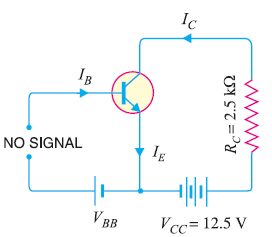Fig.12

### Solution :

The collector-emitter voltage VCE  is given by ;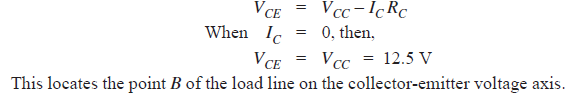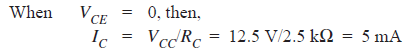This locates the point A of the load line on the collector current axis. By joining these two points, we get the d.c. load line AB as shown in Fig. 13.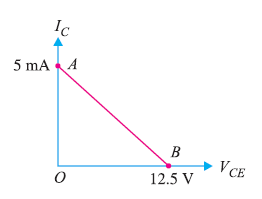Fig.13

### Q22. In the circuit diagram shown in Fig. 14, if VCC = 12V and RC = 6 kΩ,  draw the d.c. load line. What will be the Q point if zero signal base current is 20μA and β = 50 ?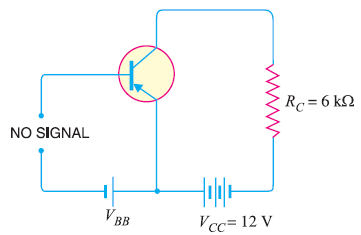Fig.14

### Solution :

The collector-emitter voltage VCE  is given by :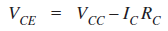When IC = 0, VCE = VCC = 12 V. This locates the point B of the load line.

When VCE = 0, IC = VCC  / RC  = 12 V/6 kΩ = 2 mA.

This locates the point A of the load line. By joining these two points, load line AB is constructed as shown in 15.Fig. 15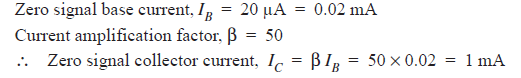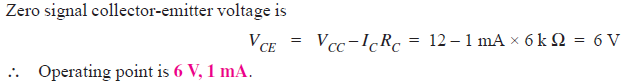Fig. 15 shows the Q point. Its co-ordinates are IC  = 1 mA and VCE= 6 V.

### Solution :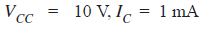(i) When collector load R= 4 k Ω , then,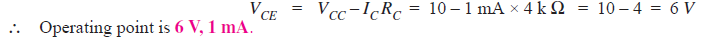(ii) When collector load R= 5 k Ω , then,### Q24. Determine the Q point of the transistor circuit shown in Fig. 16. Also draw the d.c. load line. Given β = 200 and VBE = 0.7V.Fig. 16

### Solution :

The presence of resistor RB in the base circuit should not disturb you because we can apply Kirchhoff’s voltage law to find the value of IB and hence IC (= βIB). Referring to Fig. 16 and applying Kirchhoff’s voltage law to base-emitter loop, we have,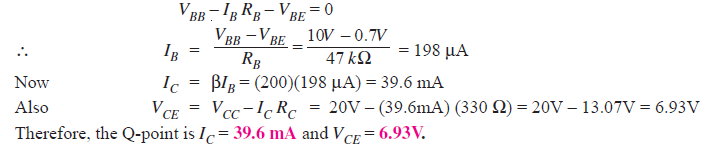In order to draw the d.c. load line, we need two end points.When IC = 0, VCE = VCC  = 20V. This locates the point B of the load line on the collector-emitter voltage axis as shown in Fig. 17.

When VCE = 0, IC = VCC / RC = 20V/330Ω = 60.6 mA. This locates the point A of the load line on the collector current axis.

By joining these two points, d.c. load line AB is constructed as shown in Fig. 17.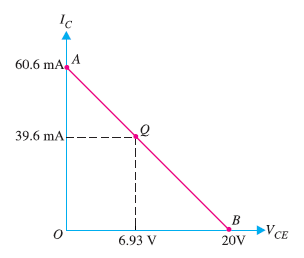Fig. 17

### Q25. Determine the Q point of the transistor circuit shown in Fig. 18. Also draw the d.c. load line. Given β = 100 and VBE = 0.7V.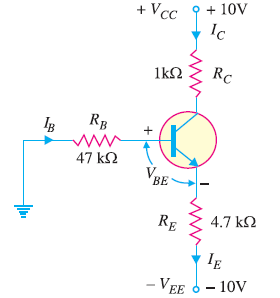Fig.18

### Solution :

The transistor circuit shown in Fig. 18 may look complex but we can easily apply Kirchhoff’s voltage law to find the various voltages and currents in the circuit.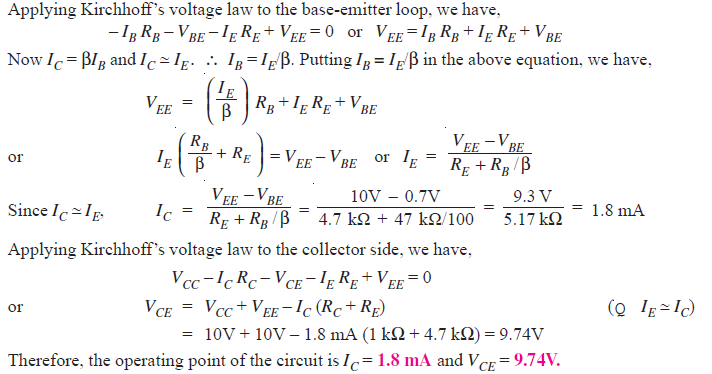The d.c. load line can be constructed as under :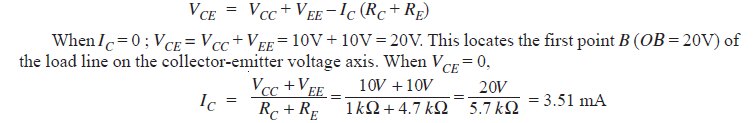This locates the second point A (OA = 3.51 mA) of the load line on the collector current axis. By joining points A and B, d.c. load line AB is constructed as shown in Fig. 19.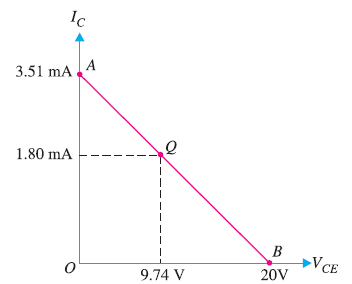Fig.19

### Solution :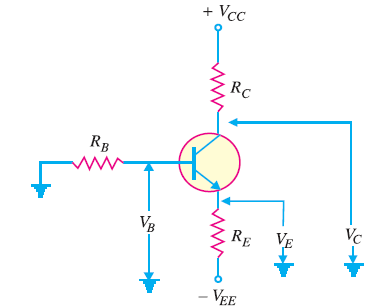Fig.20

Referring  to Fig. 20:

(i) The emitter voltage w.r.t. ground is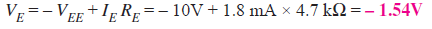(ii) The base voltage w.r.t. ground is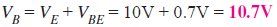(iii) The collector voltage w.r.t. ground is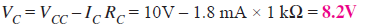### Solution :

Change in collector-emitter voltage is :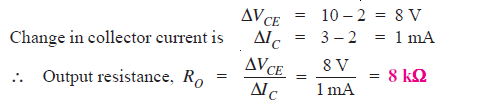### Solution :

Change in base-emitter voltage is :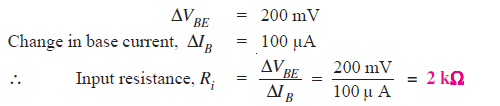###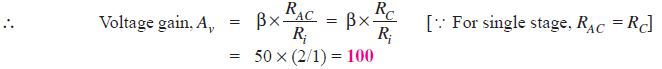### Q30. Find IC(sat) and VCE(cutoff)  for the circuit shown in Fig. 21.Fig.21

### Solution :

As we decrease RB, base current and hence collector current increases. The increased collector current causes a greater voltage drop across RC ; this decreases the collector-emitter voltage.

Eventually at some value of RB, VCE  decreases to Vknee . At this point, collector-base junction is no longer reverse biased and transistor action is lost.

Consequently, further increase in collector current is not possible. The transistor conducts maximum collector current or we can say the transistor is saturated.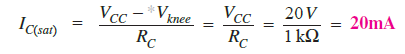As we increase RB, base current and hence collector current decreases. This decreases the voltage drop across RC. This increases the collector-emitter voltage. Eventually, when IB = 0, the emitter-base junction is no longer forward biased and transistor action is lost.

Consequently, further increase in VCE is not possible. In fact, VCE now equals to VCC.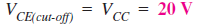Fig. 22 shows the saturation and cut off points. Incidentally, they are end points of the d.c. load line.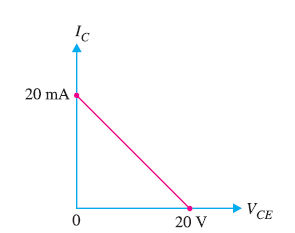Fig. 22

### Q31. Determine the values of VCE(off) and IC(sat) for the circuit shown in Fig. 23.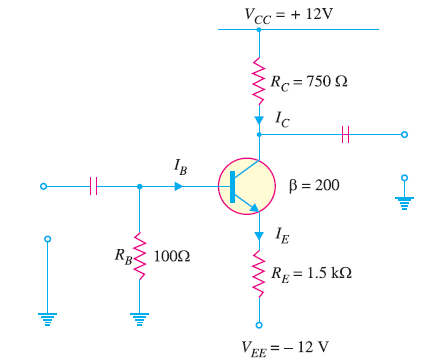Fig. 23

### Solution :

Applying Kirchhoff’s voltage law to the collector side of the circuit in Fig. 23, we have ,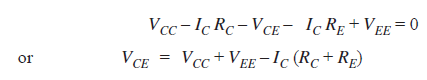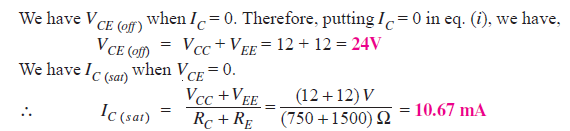### Q32. Determine whether or not the transistor in Fig. 24 is in stauration. Assume Vknee = 0.2V.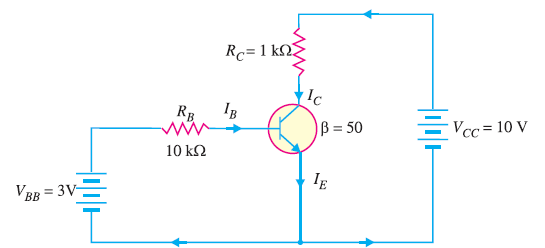Fig.24

### Solution :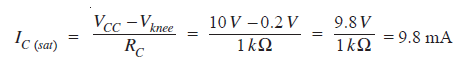Now we shall see if IB is large enough to produce IC(sat).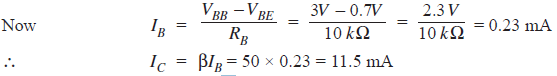This shows that with specified β, this base current (= 0.23 mA) is capable of producing IC greater than IC(sat). Therefore, the transistor is saturated. In fact, the collector current value of 11. 5 mA is never reached. If the base current value corresponding to IC(sat) is increased, the collector current remains at the saturated value (= 9.8 mA).

### Q33. Is the transistor in Fig. 25 operating in saturated state ?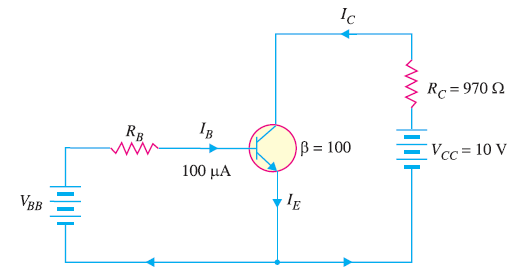Fig.25

### Solution :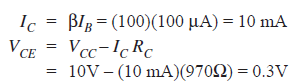Let us relate the values found to the transistor shown in Fig. 26.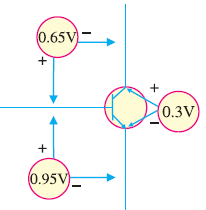Fig.26

As we can see, the value of VBE is 0.95V and the value of VCE = 0.3V.

This leaves VCB of 0.65V (Note that VCE = VCB + VBE).

In this case, collector–base junction (i.e., collector diode) is forward biased as is the emitter-base junction (i.e., emitter diode). Therefore, the transistor is operating in the saturation region.

### Q34. For the circuit in Fig. 27, find the base supply voltage ( VBB) that just puts the transistor into saturation. Assume β = 200.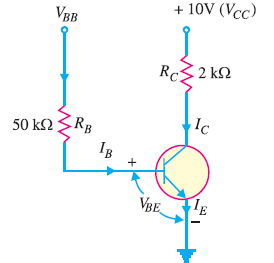Fig.27

### Solution :

When transistor first goes into saturation, we can assume that the collector shorts to the emitter (i.e. VCE = 0) but the collector current is still β times the base current.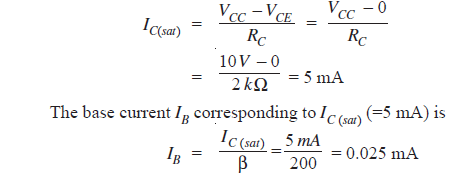Applying Kirchhoff’s voltage law to the base circuit, we have,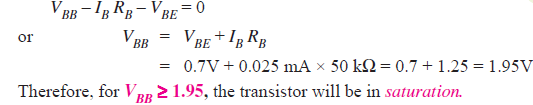### Q35. Determine the state of the transistor in Fig. 28 for the following values of collector resistor : (i) RC = 2 kΩ (ii) RC = 4 kΩ (iii) RC = 8 kΩ.Fig. 28

### Solution :

Since IE does not depend on the value of the collector resistor RC, the emitter current ( IE) is the same for all three parts.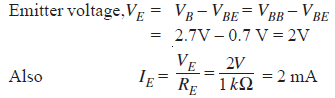(i) When RC = 2 kΩ

Suppose the transistor is active.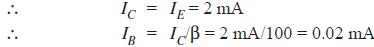Since VC (= 6V) is greater than VE (= 2V), the transistor is active. Therefore, our assumption that transistor is active is correct.

(ii) When RC = 4 kΩ

Suppose the transistor is active.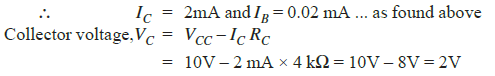Since VC = VE, the transistor is just at the edge of saturation.
We know that at the edge of saturation, the relation between the transistor currents is the same as in the active state. Both answers are correct.

(iii) When RC = 8 kΩ

Suppose the transistor is active.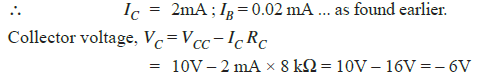Since VC < VE, the transistor is saturated and our assumption is not correct

### Q36. In the circuit shown in Fig. 29, VBB is set equal to the following values: (i) VBB = 0.5V (ii) VBB = 1.5V (iii) VBB = 3V. Determine the state of the transistor for each value of the base supply voltage VBB.Fig.29

### Solution :

The state of the transistor also depends on the base supply voltage VBB

(i) For VBB = 0.5V

Because the base voltage VB (= VBB = 0.5V) is less than 0.7V, the transistor is cut-off.

(ii) For VBB = 1.5V

The base voltage VB controls the emitter voltage VE which controls the emitter current IE.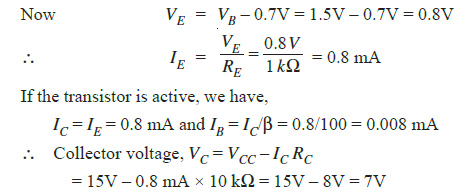Since VC > VE, the transistor is active and our assumption is correct.

(iii) For VBB  = 3V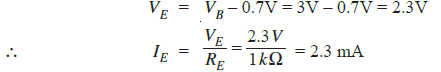Assuming the transistor is active, we have,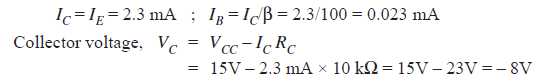Since V< VE, the transistor is saturated and our assumption is not correct.

### Q38. For the circuit shown in Fig. 30, find the transistor power dissipation. Assume that β = 200.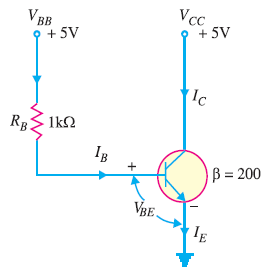Fig.30

### Solution :

The transistor is usually used with a resistor RC connected between the collector and its power supply VCC as
shown is Fig.31.

The collector resistor RC serves two purposes. Firstly, it allows us to control the voltage VC at the collector.
Secondly, it protects the transistor from excessive collector current IC and, therefore, from excessive power dissipation.

Referring to Fig. 31 and applying Kirchhoff’s voltage law to the base side, we have,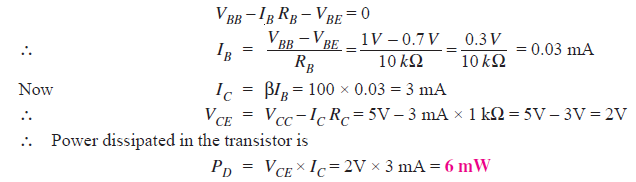### Q40. The transistor in Fig.32 has the following maximum ratings : PD (max) = 800 mW ; VCE(max) = 15V ; IC(max) = 100 mA. Determine the maximum value to which VCC can be adjusted without exceeding any rating. Which rating would be exceeded first ?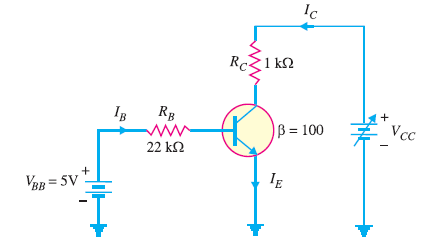Fig.32

### Solution :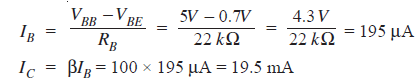Note that Iis much less than IC(max) and will not change with VCC. It is determined only by IB and β. Therefore, current rating is not exceeded.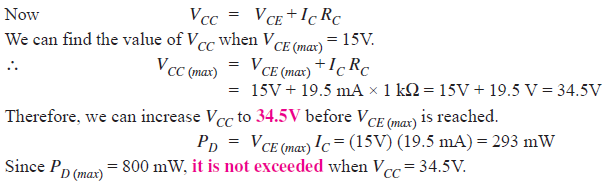If base current is removed causing the transistor to turn off, VCE(max) will be exceeded because the entire supply voltage VCC will be dropped across the transistor.